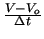Next: 2.3.3 Devices Up: 2.3 Constitutive Relation Formulation Previous: 2.3.1 Thermal Simulation

## 2.3.2 Time Discretization

Capacitances and inductances are considered in the time domain only and the derivatives are discretized using finite difference methods. The most common difference method is the backward Euler scheme which is an implicit first-order method. For example, for a linear capacitor the time derivative of the current I = C . dV/dt is represented in terms of the backward Euler scheme as

 I = I(t) = C .(2.28)

with Vo being the voltage of the last time step.

Tibor Grasser
1999-05-31